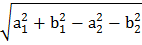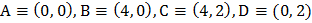# If the equation of the locus of a point equidistant  from the pointsandis, then the value ofis a)b)c)d)## Question ID - 53552 :- If the equation of the locus of a point equidistant  from the pointsandis, then the value ofis a)b)c)d)3537

(d)

Letbe the point on the locus. Then by the given conditions,(i)

Also, sincelies on the given locus, therefore(ii)

Comparing Eqs. (i) and (ii), we getNext Question :rectangle, where, undergoes the following transformations successively: (i), (ii), (iii). The final figure will be a) A square b) A rhombus c) A rectangle d) A parallelogram